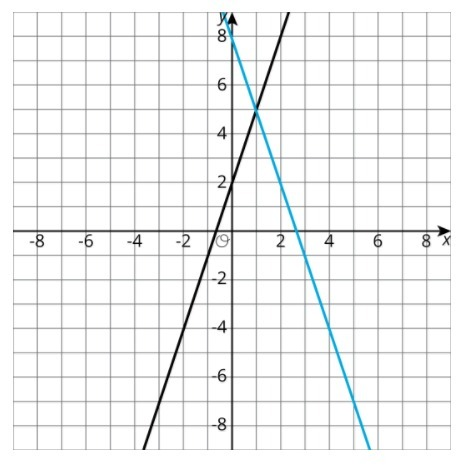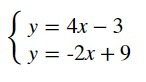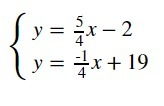PSABM5X5 - Practice---Acc7.5 Lesson 14 Solving Systems of Equations (8.EE.C.8, 8.EE.C.8.a)
Part A)

Write an equation for the black line shown:Complete the equation below

y = _______________

Part B)

Write an equation for the blue line.

Complete the equation below

y = _______________

Part C)

Describe how to find the solution to the corresponding system by looking at the graph.

Part D)

Describe how to find the solution to the corresponding system by using the equations.

The solution to a system of equations is (5,-19). Choose two equations that might make up the system.

1. y = -3x - 6
2. y = 2x - 23
3. y = -7x + 16
4. y = x - 17
5. y = -2x - 9

Select all that apply:

Solve the system of equations:(x,y)

with no spaces

Solve the system of equations:(x,y)

with no spaces

Part A)

Here is an equation:Solve the equation by using the distributive property first.

Part B)

Solve the equation without using the distributive property.